# 定义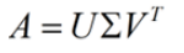A是一个m*n的矩阵，那么得到的U是一个m * m的方阵（里面的向量是正交的，称为左奇异向量），Σ是一个m * n的矩阵（除了对角线的元素外，其它都是0，对角线上的元素称为奇异值），VT是一个n * n的矩阵（里面的向量也是正交的，称为右奇异向量）。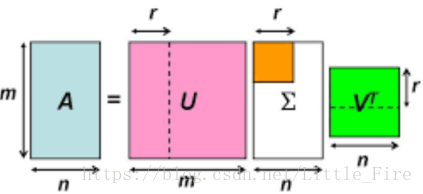# 右奇异向量求解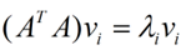# 左奇异向量和奇异值的求解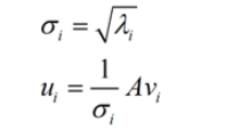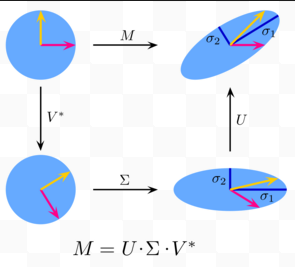# python代码实现

``````m = 4
n = 3
A = np.random.randint(-10,10,(m, n)).astype(float)

# np.dot(A.T, A)的特征向量构成的矩阵为rvecs，需要注意的是rvecs的每个行向量为特征向量
lvecs, vals, rvecs = np.linalg.svd(A)

# 验证是否正确
# *表示只对固定位置的数相乘
np.allclose(A, np.dot(lvecs[:, :n] * vals, rvecs))

# 将s转化为奇异值矩阵
smat = np.zeros((m, n))
smat[:n, :n] = np.diag(vals)
np.allclose(A, np.dot(lvecs, np.dot(smat, rvecs)))``````